##### Circuit Analysis For Dummies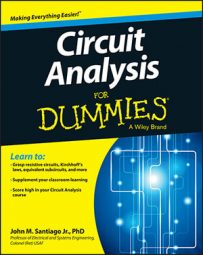The impulse function, also known as a Dirac delta function, helps you measure a spike that occurs in one instant of time. Think of the spiked impulse function (Dirac delta function) as one that’s infinitely large in magnitude and infinitely thin in time, having a total area of 1. Impulse forces occur for a short period of time, and the impulse function allows you to measure them.

Visualize the impulse as a limiting form of a rectangular pulse of unit area. Specifically, as you decrease the duration of the pulse, its amplitude increases so that the area remains constant at unity. The more you decrease the duration, the closer the rectangular pulse comes to the impulse function.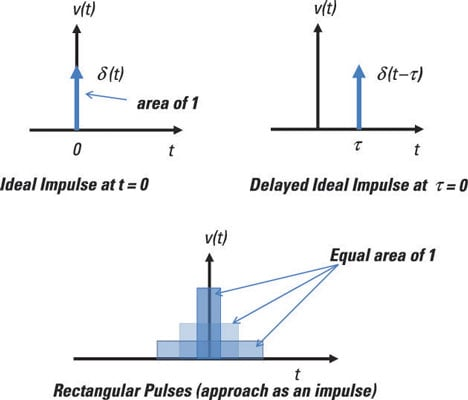The bottom diagram here shows the limiting form of the rectangular pulse approaching an impulse.

So what’s the practical use of the impulse function? By using the impulse as an input signal to a system, you can reveal the output behavior or character of a system. After you know the behavior of the system for an impulse, you can describe the system’s output behavior for any input.

Why is that? Because any input is modeled as a series of impulses shifted in time with varying heights, amplitudes, or strengths.

Here’s the fancy pants description of the impulse function: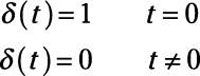## Identify impulse functions in the day-to-day

Some physical phenomena come very close to being modeled with impulse functions. One example is lightning. Lightning has lots of energy and occurs in a short amount of time. That fits the description of an impulse function.

An ideal impulse has an infinitely high amplitude (high energy) and is infinitely thin in time. As you drive through a lightning storm, you may hear a popping noise if you’re tuned in to a radio weather station. This noise occurs when the energy of the lightning interferes with the signal coming from the radio weather station.

Another example of a real-world impulse function is a bomb. A powerful bomb has lots of energy occurring in a short amount of time. Similarly, fireworks, including cherry bombs, produce loud noises — audio energy — that occur as a series of popping noises having short durations.

This mathematical description says that the impulse function occurs at only one point in time; the function is zero elsewhere. The impulse here occurs at the origin of time — that is, when you decide to let t = 0 (not at the beginning of the universe or anything like that).

The top-left diagram here shows an ideal unit impulse function having a large amplitude with a short duration.You can describe the area of the impulse function as the strength of the impulse: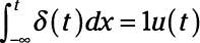At time t = 0, the area is a constant having a value of 1; and before t = 0, the area is equal to 0. The integration of the impulse results in another funky function, u(t), called a step function. You can view the impulse as a derivative of the step function u(t) with respect to time: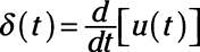What these two equations tell you is that if you know one function, you can determine the other function.

## Change the strength of the impulse

The figure shows an impulse with an area (or strength) equal to 1. To have a different area or strength K, you can modify the impulse: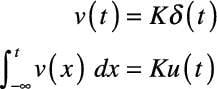The area under the curve is given by strength K. The result of integrating the impulse leads you to another step function with amplitude or strength K.

## Delay an impulse

Impulses can be delayed. Analytically, you can describe a delayed impulse that occurs later, say, at time τ: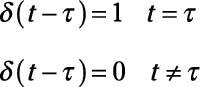This equation says the impulse occurs only at a time later τ and nowhere else, or it’s equal to 0 at time not equal to τ. You see a delayed impulse in the top-right diagram shown here.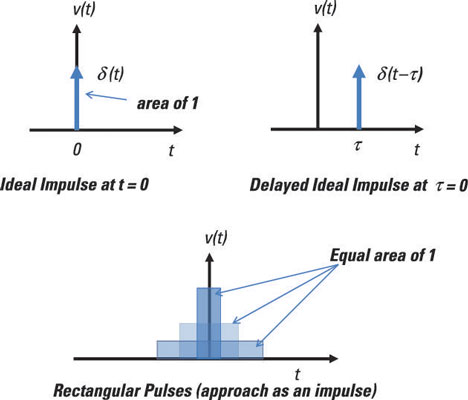For a numerical example, let an impulse having a strength of 10 occur at delayed time τ = 5. You can describe the delayed impulse as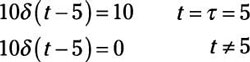The equation says that the impulse, which has strength K = 10, occurs only at a time τ = 5 later and that the impulse occurs nowhere else. In other words, the impulse is equal to 0 when time is not equal to 5.

## Evaluate impulse functions with integrals

Assuming x(t) is a continuous function that’s multiplied by a time-shifted (or delayed) impulse, the integral of the product is expressed and evaluated as follows: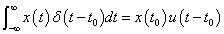You do this evaluation only where the impulse occurs — at only one point and nowhere else. The preceding equation sifts out or selects the value of x(t) at time equal to t0. This integration is one of the easiest integrations you’ll encounter.

Here’s a simple numerical example with x(t) = 5t2 + 3t + 6 and t0 = 5: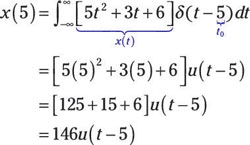Pretty funky way to integrate analytically, huh? The integration leads to a delayed (or time-shifted) step function (or constant) starting at a delayed time of t0 = 5.

You can model any smooth function x(t) as a series of delayed and time-shifted impulses in the following way: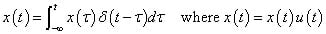This equation says you can break up any function x(t) into a sum of a whole bunch of delayed impulse functions with different strengths. The value of the strength is simply the function x(t) evaluated where the shifted impulse occurs at time τ or t.